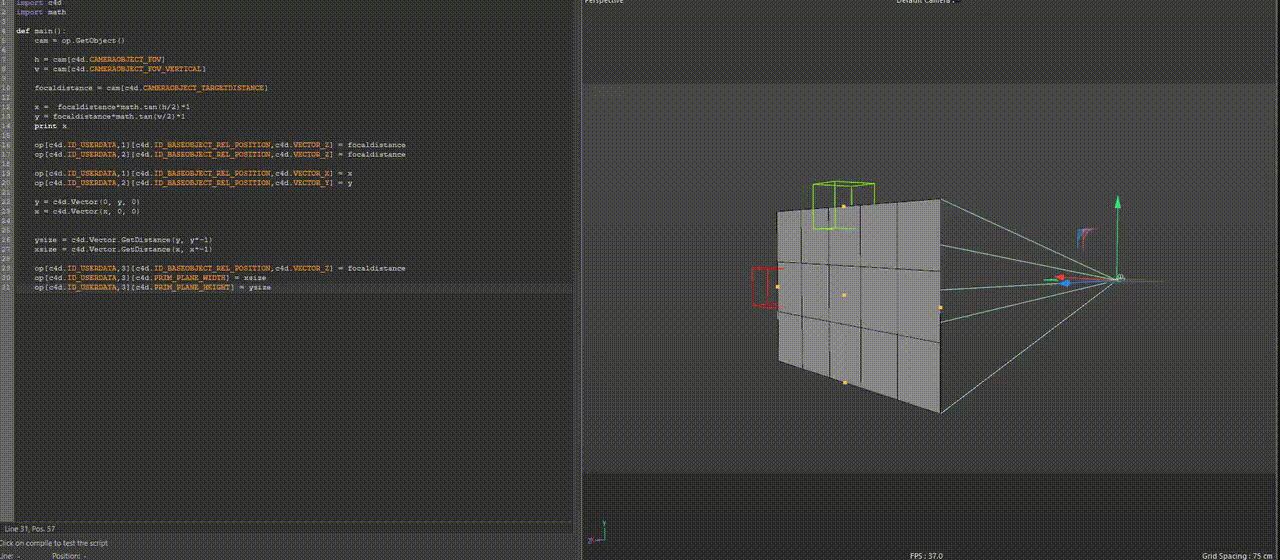# Solved Get height and width (cm) of a camera focus area

I would like to know if it is possible to get the height and length of field of view in cm individually from a camera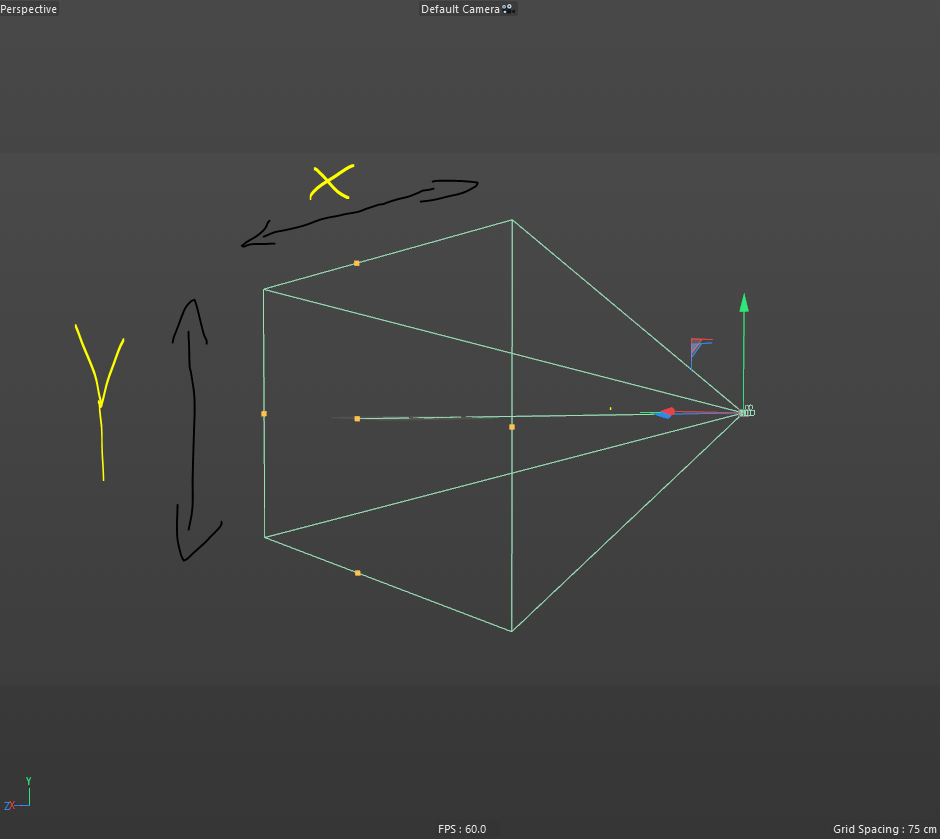I see that the camera gives me values in degrees, but I need to have them in CM
is there any way I can get these values without having to do complex calculations based on Field Of View and Focus distance?

Hello,

Thank you for reaching out to us. I am not sure if I do understand the question correctly.

I see that the camera gives me values in degrees, but I need to have them in CM

With the possibility of stating here the obvious, let me spell out some things, so that we are on the same page. The dimensions of the view frame are usually measured in pixels, i.e., the dimensions of the photo or rendered image. You can convert that into a measure of distance, e.g., cm, but for that you need to define a pixel density, the DPI. The rest is just multiplication when you know how many pixels should fit into an inch.

The pyramid shown in the viewport is just a visual aid to represent the camera frustrum. There is normally no physical quality to it. When you want to calculate the physical dimensions of the view frame, as if it would be projected onto the plane that contains the focal point and is normal to the camera z-axis, i.e., the flat plane which is in front of the frustrum gizmo, then this is just an exercise in quite simple trigonometry. There is no method in our API which would do that for you, as this is a rather uncommon thing to calculate I would say. And this would usually be a value in meters and not centimeters for the common Cinema 4D scene, as the default value of the focus distance of a C4D camera is 2m.

So, when we know the length of the focal point vector, the adjacent (b) in the triangle, and we know two angles, the horizontal and vertical field of view, then we can calculate the opposite (a), i.e., what you seem to be after.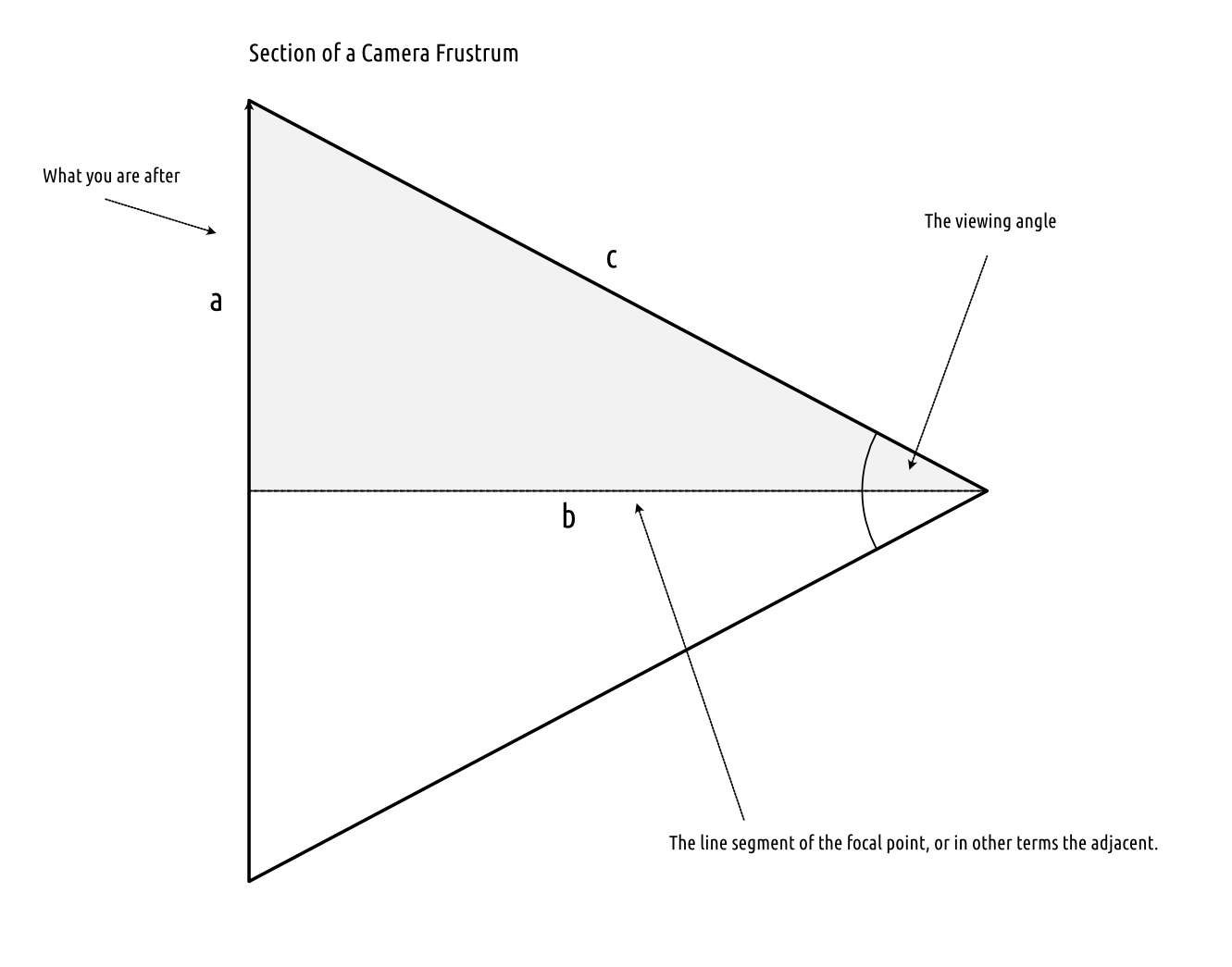The opposite and the adjacent are defined over the tangent of the input angle:You then just must remember that you must cut the viewing angle in half, since we only calculate a subsection of it and then multiply the result of the opposite (a) times two, since you are after the full length. You then must do that for both the horizontal and vertical section and it will give you the length of x and y in your image.

Cheers,
Ferdinand

MAXON SDK Specialist
developers.maxon.net

maybe it got a little confusing, i mean you need to get the radio aspect size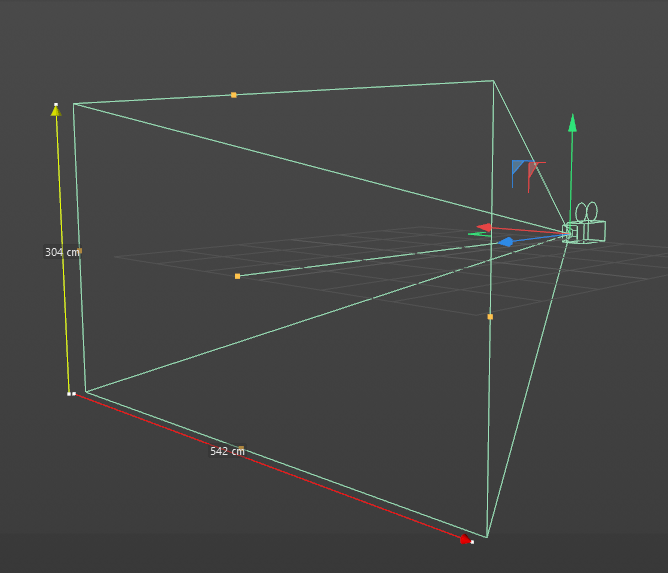it gives me in degrees...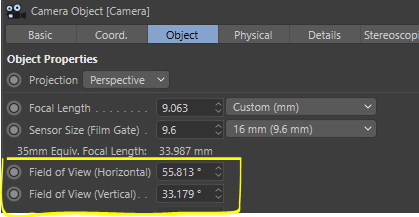I imagine it's something super simple.. but I didn't find anything

Hello @pyxelrigger,

maybe it got a little confusing, i mean you need to get the radio aspect size

There is obviously some language barrier between us here, but this does not make it much clearer to me. What do you mean by "radio aspect size"? I assume you mean "ratio aspect size", but that does not make things much clearer. The aspect ratio for a camera frustrum and the view frame are the same, no matter which values you take. Image width over image height in pixels, horizontal frustrum angle over vertical frustrum angle, the X and Y in your images, they are all in the same relation. The easiest way to obtain that ratio is to use the horizontal and vertical render resolution.it gives me in degrees...

You cannot just convert degrees into a unit of distance, as there are infinite points along a ray where you could measure the distance to another point on another ray. But you can do it as lined out in my last posting with the focal point, you should read it :) The math is not that hard, and this is a programming forum, I cannot carry you the whole way. Show us some code, and when you are then still struggling, I will help you.

To make this clear, there are two triangles in a camera frustrum: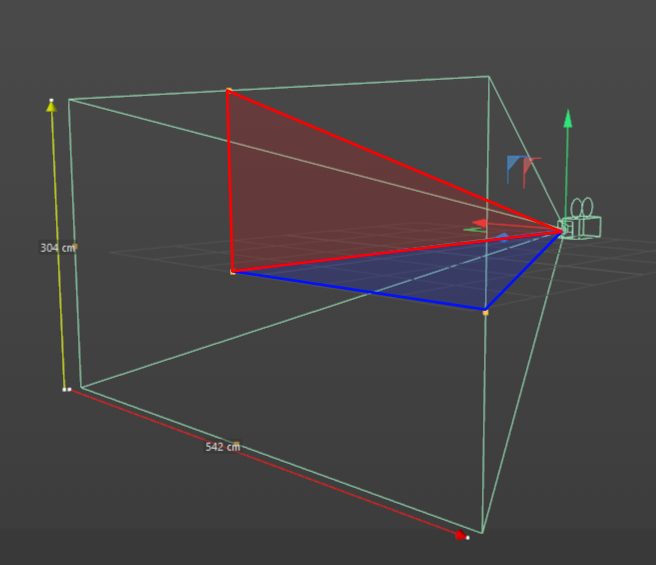The reasoning in my last posting shows you how to calculate the values you are after with the help of the focal point and an angle. With this trigonometric relationyou can derive that `x = focal_distance * tan(horizontal_viewing_angle / 2) * 2` for example, but there are some hoops to jump through along the way.

Cheers,
Ferdinand

MAXON SDK Specialist
developers.maxon.net

Hmm thank you!! It's not as difficult as I imagined I just had to make a change in the formula for some case, I used:

``````x =  focaldistance*math.tan(h/2)*1
``````